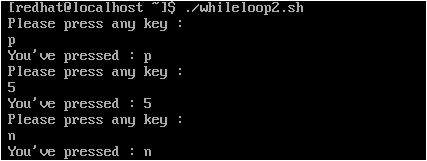## Saturday, March 3, 2018

### Learn Shell Scripting -- Part 2

Conditional operations using “if…else…fi” statement

We could use “if” statement when it is required to perform a conditional based operation. So, if the condition is true then process a set of commands, otherwise, process some other commands/action. The syntax of this is:

if [ <conditional statement> ]
then
< statements >    <--------- these statements gets executed if condition is true.
else
< statements >   <--------- these statements gets executed if condition fails.
fi

There is multiple conditional test mode in “if” by using “elif” as shown below which is also called as “nested if”:

if [ <conditional statement> ]
then
< statements >   <--------- these statements gets executed if initial condition is true.
elif [ <conditional statement> ]
then
< statements >   <--------- these statements gets executed if 'elif' condition is true.
else
< statements >   <--------- these statements gets executed if none of above conditions fails.
fi

We could also use “if” condition without “else” for simple conditional evaluation as shown below:

if [ <conditional statement> ]
then
< statements >
fi

So, ground rule of using "if" statement is that it should always ends with "fi" & after conditional statement there should be "then" which usually on next line as shown above.

Practice Example 1:
--------------------------

Let’s create a simple script file which should read input from user and prints a message based on number entered. Here I’m going to consider the shell standard input (0), standard output (1) & standard error (2) numerical values and accordingly print message using “if...elif...else...fi” statement. The sample script is shown below: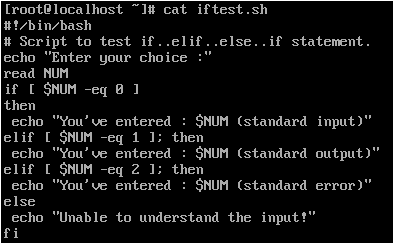Now, we’d run the script and test.NOTE: As you could see in the above script file “iftest.sh”, I’ve used
“elif [ \$NUM -eq 1 ]; then”
statement which reduces the number of lines by combining 'condition' and 'then' operator separated by “;”  on same line.

The "read" commands reads input from keyboard and stores in a variable.

Conditional operations using "case...esac" statement

When there is a need to test for multiple conditions then instead of using “nested if-loops” it is a good practice to use “case…esac” as shown below.

case <variable> in
pattern1)
< statement > ;; <--------- these statements gets executed if 'variable' matches to 'pattern1'
pattern2)
< statement > ;; <--------- these statements gets executed if 'variable' matches to 'pattern2'
pattern3)
< statement > ;; <--------- these statements gets executed if 'variable' matches to 'pattern3'
*)
< statement > <--------- these statements gets executed if 'variable' matches to none of the patterns
esac

NOTE: Each pattern statements should end with ";;" and end of the case statement should terminate with "esac".

Practice Example 1:
--------------------------

I’d use the same example as stated above (used in nested if-loop) in this script to demonstrate usage of “case” statement. The script should be as shown below: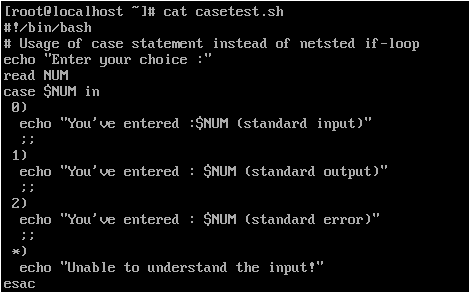Let’s execute the script and test if that works as expected: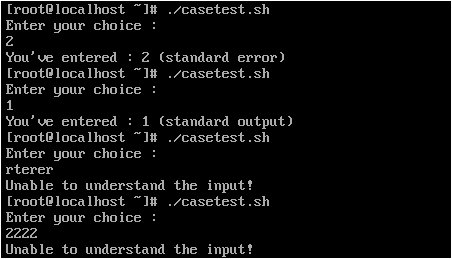As you could see the variable "NUM" is evaluated and depending on number entered the respective case statements gets processed.

- If NUM is 0 then it would print "You've entered : 0 (standard input)”.

- if NUM is 1 then it would print "You've entered : 1 (standard output)”.

- If NUM is 2 then it would print "You've entered : 2 (standard error)”.

- If NUM is other than 0 or 1 or 2 then it would print "Unable to understand the input!".

Practice Example 2:
--------------------------

In this example, I’d create a shell script which could print out Linux distribution vendor name depending on the user input. So, if user enters “rhel” then it would print the vendor name as “Red Hat” as shown below (this is just an example):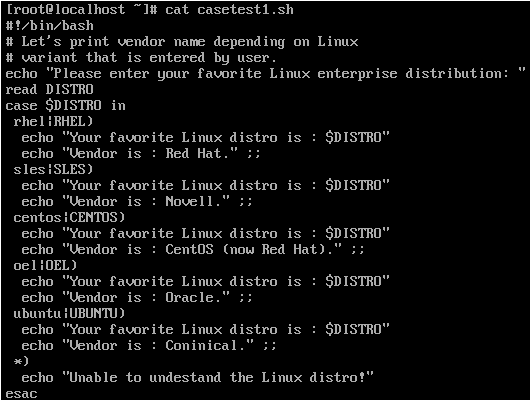Please remember to set “execute” bit in each example script and then run the script. You might have noticed that I've used two words in each case loop here, so, it could match out either of the word (lower-case|upper-case). This is how it prints data when executed: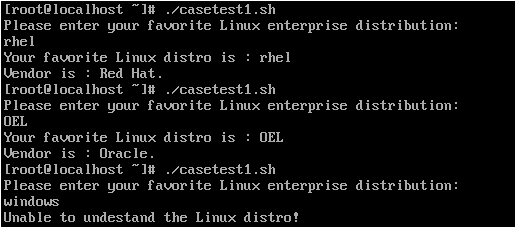Usage of “for” loop (iteration) statement

Whenever there is a necessity to repeat a set of statements/commands then we could use “for” loop. This is considered as iteration.

The syntax of “for” loop is as stated below:

for <VAR> in 1 2 3 … N <--------- VAR is the variable & "1 2 3 ... N" are respective values assigned to VAR
do                                                                                                                                       in each loop
<command1> <--------- these commands would gets
<command2> <--------- executed in each loop
done

Practice Example 1:
--------------------------

So, let’s print 5 (from 1 to 5) numbers using “for” loop now and check how this works. Create a script file "forloop1.sh" using "vi" or any other editor of your choice as shown below: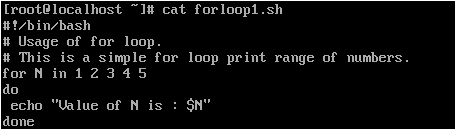Now, let's run the script file and see what it prints: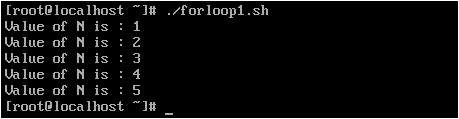Practice Example 2:
--------------------------

Likewise let’s print all file names under current working directory one by one and their attributes (like date of creation, size, owner etc.,) using for loop. So, the script should be like this: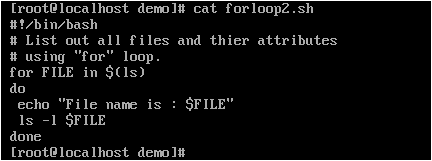Set the execute bit on this file and run the script and check what it prints on terminal. You should get to see similar output as shown here: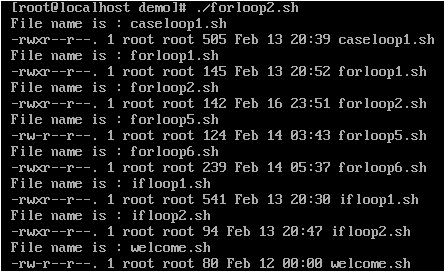In the above script file "forloop2.sh" I've used the "ls" command output which in tern is file/directory names which gets passed to the "FILE" variable one-by-one in each loop, and then prints the name of those file/directory and their attributes.

Practice Example 3:
--------------------------

The “for” loop could be used to set a range of values enclosed in curly braces to be iterated as shown below: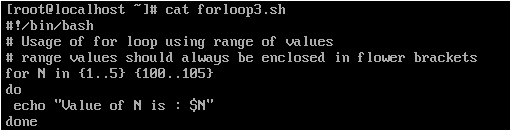As you could see in the above example, I've used two range of values separated each by a space. So, each range gets evaluated separately and we could specify values as shown here. Let's execute the script and see what it prints (it should print first list of values of 1..5 and second list with values from 100..105):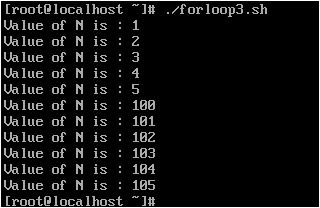Practice Example 4:
--------------------------

Likewise Bash v4.0+ has inbuilt support for setting up a step value using
{ START..END..INCREMENT } syntax as demonstrated in the below snap to print range of numbers which gets incremented in each loop (example: to print only odd numbers from 1 to 10):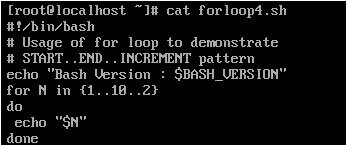After creating the script, let's set the execute bit and run it. Here, 'N' would initially start from 1 and in each loop it would increment by 2 till it reaches 10 and then it terminates the loop.

The output should be like this: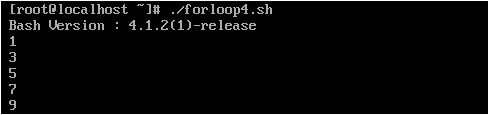Usage of “three-expression for loop”

This format is inherited from popular “C” language style which is “initialization”, “testing”, “counting expression” as shown in the below syntax:

for (( EXP1; EXP2: EXP3 ))
do
command1
command2
done

In the above syntax, EXP1 would do the loop initialization (gets executed only once during the beginning of loop), EXP2 would perform test evaluation and if it passes then commands inside the "do.... done" labels would get executed & EXP3 would perform numerical operation in each loop.

Practice Example 5:
--------------------------
Lets' print out numbers from 1 to 5 using this method now. So, create a script file "forloop5.sh" as shown below and execute it.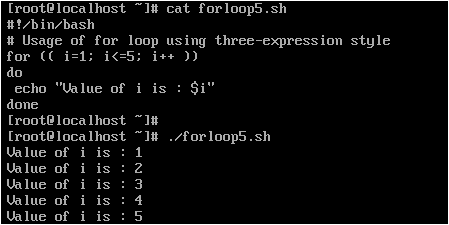> here “i=1” is for initialization, “i<=5” is testing code & “i++” is the counting expression (i=i+1).

Practice Example 6:
--------------------------

Let’s print a pyramid of numbers using “three-expression for loop” and then reverse print the pyramid. In this example, I’d print only 1..10 numbers in pyramid style as shown in the below “forloop6.sh” file:

So, the pyramid should look similar to this one:

1
1 2
1 2 3
1 2 3 4
1 2 3
1 2
1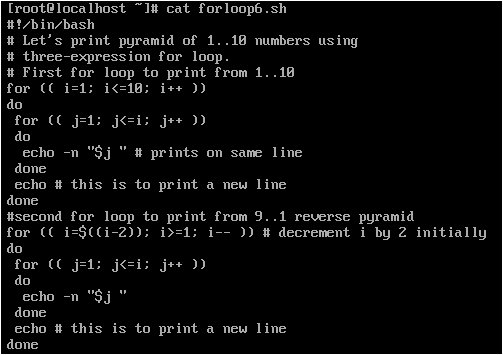When executed this would print the pyramid of numbers from 1..10 and from 9..1 in reverse as shown below: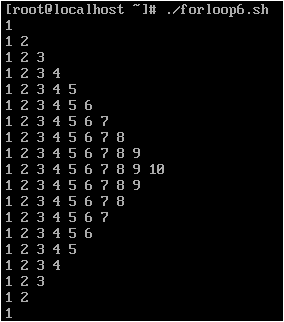Usage of “while” loop

Like “for” loop there is “while” loop which is normally used to repeat some commands until a condition is met or not met whichever suitable. The syntax of the “while” loop is as shown below:

while [ condition ] <---------- loop runs if the condition is met
do
<command1>
<command1>
done

Practice Example 1:
--------------------------

Let’s create a simple shell script file to print 10 numbers one by one using “while” loop. The script file "whileloop1.sh" should be as shown below: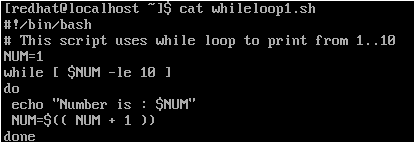It should produce the below output when ran: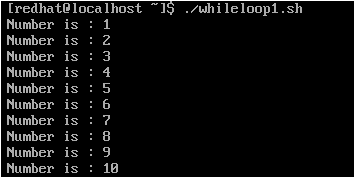NOTE: The "while" loop always terminates with "done" statement.

Practice Example 2:
--------------------------

Another example of “while” loop running until user hits “n” key. So, create a shell script file "whileloop2.sh" as shown below: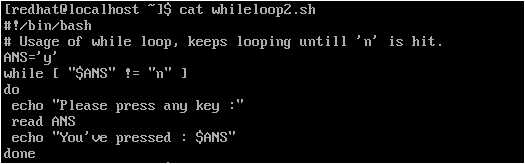Let’s execute the script and check if that works as expected. Yes, it is working as shown below: